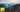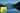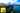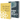# Gradient Descent with Vectorization in JavaScript

by Robin WieruchA recent article gave an introduction to the field of machine learning in JavaScript by in a univariate regression problem. It used plain mathematical expressions and thus made use of the unvectorized implementation of gradient descent and the cost function. This article takes it one step further by implementing a vectorized gradient descent in JavaScript. It will guide you through the process step by step. In the end, you will find the whole source code.

I highly recommend to take the Machine Learning course by Andrew Ng. This article will not explain the machine learning algorithms in detail, but only demonstrate their usage in JavaScript. The course on the other hand goes into detail and explains these algorithms in an amazing quality. At this point in time of writing the article, I learn about the topic myself and try to internalize my learnings by writing about them and applying them in JavaScript. If you find any parts for improvements, please reach out in the comments or create a Issue/Pull Request on GitHub.

## Why and what is vectorization?

Gradient descent by nature is an iterative process. You take a number of iterations and let gradient descent do its thing by adjusting the theta parameters according to the partial derivative of the cost function. Thus there are a bunch of for loops in the algorithm when using the unvectorized implementation.

`for (let i = 0; i < ITERATIONS; i++) {  for (let j = 0; j < m; j++) {    thetaZeroSum += hypothesis(x[j]) - y[j];    thetaOneSum += (hypothesis(x[j]) - y[j]) * x[j];  }   thetaZero = thetaZero - (ALPHA / m) * thetaZeroSum;  thetaOne = thetaOne - (ALPHA / m) * thetaOneSum;}`

There are a couple of shortcomings for the unvectorized implementation. First, extending the training set from a univariate to a multivariate training set. It wouldn't be too easy anymore to consider all the features n in matrix x. In the example x is only an array, but in a multivariate training set it would be a matrix. Second, in any event there needs to be a loop over the size m of the training set. It is computational inefficient, because it needs an iterative process to compute each theta parameter for each data point in the training set.

Isn't there a way to compute all theta parameters in one mathematical expression using the training set with all its data points m and all its features n and on the other hand the labels y? That's the point where come into play. They solve all the shortcomings from before: the implementation becomes simpler for multivariate training sets and it becomes computational efficient by omitting the loops.

## Vectorization in JavaScript

Imagine a training set about houses with the size of m (m = 50, each row a house) with features n (n = 1, size of a house). It can be expressed in a matrix. Furthermore, the label y (price of a house) can be expressed in a matrix too. If you would have a function in JavaScript, which arguments would have the whole training set in a matrix, you could split up the training set in the unlabeled training set (matrix X) and the labels (matrix y).

`function init(matrix) {   // Part 0: Preparation   let X = math.eval('matrix[:, 1]', {    matrix,  });  let y = math.eval('matrix[:, 2]', {    matrix,  });   ...}`

That way, you have already vectorized your data. Everything is represented in a matrix now. Next you can apply matrix operations rather than looping over the data. The concept will be used for the cost function and gradient descent in the next parts. Don't worry too much about the code yet, because you will get access to it in the end of the article to play around with it. Keep in mind to take the machine learning course on Coursera to learn about the algorithms yourself and revisit those articles of mine to implement them in JavaScript. Now, let's start by implementing the vectorized cost function.

## Vectorized Cost Function in JavaScript

Before implementing the cost function in JavaScript, the matrix X needs to add an intercept term. Only this way the matrix operations work for theta and matrix X.

`function init(matrix) {   // Part 0: Preparation   let X = math.eval('matrix[:, 1]', {    matrix,  });  let y = math.eval('matrix[:, 2]', {    matrix,  });   let m = y.length;   // Part 1: Cost   // Add Intercept Term  X = math.concat(math.ones([m, 1]).valueOf(), X);}`

Now, let's implement the cost function. It should output the cost depending on input matrix X, output matrix y and the eventually trained parameters theta. The cost depends on theta, because X and y stay fixed as you have prepared those matrices before already. In addition, theta will be represented in a matrix to enable it for matrix operations. In the beginning, the theta parameters will have a random initial value such as -1 and 2 and thus the hypothesis being `h(x) => -1 + 2 * x`. No worries, they will be trained later on. Now they are only used to demonstrate the cost function.

`function init(matrix) {   ...   // Part 1: Cost   // Add Intercept Term  X = math.concat(math.ones([m, 1]).valueOf(), X);   let theta = [[-1], ];  let J = computeCost(X, y, theta);} function computeCost(X, y, theta) {  ...   return J;}`

The cost function returns the cost J. Now it needs only to compute the cost by using matrix operations. First, you can express the hypothesis with matrix multiplication by multiplying the matrix X of the training set with the parameters matrix theta.

`function computeCost(X, y, theta) {  let m = y.length;   let predictions = math.eval('X * theta', {    X,    theta,  });   ...   return J;}`

Second, the squared errors need to be computed too. It can be done in a element wise matrix operation to the power of 2.

`function computeCost(X, y, theta) {  let m = y.length;   let predictions = math.eval('X * theta', {    X,    theta,  });   let sqrErrors = math.eval('(predictions - y).^2', {    predictions,    y,  });   ...   return J;}`

And last but not least, computing the cost with the squared errors and the training set size m.

`function computeCost(X, y, theta) {  let m = y.length;   let predictions = math.eval('X * theta', {    X,    theta,  });   let sqrErrors = math.eval('(predictions - y).^2', {    predictions,    y,  });   let J = math.eval(`1 / (2 * m) * sum(sqrErrors)`, {    m,    sqrErrors,  });   return J;}`

That's it. Now you are able to compute the cost depending on your parameters theta. When using gradient descent, the cost have to decrease with each iteration. You can compute a couple of costs by using random theta parameters before you have trained them.

`function init(matrix) {   ...   // Part 1: Cost   // Add Intercept Term  X = math.concat(math.ones([m, 1]).valueOf(), X);   let theta = [[-1], ];  let J = computeCost(X, y, theta);   console.log('Cost: ', J);  console.log('with: ', theta);  console.log('\n');   theta = [, ];  J = computeCost(X, y, theta);   console.log('Cost: ', J);  console.log('with: ', theta);  console.log('\n');}`

In the next part, you will implement the vectorized gradient descent algorithm in JavaScript.

## Vectorized Gradient Descent in JavaScript

As you know, the gradient descent algorithm, takes a . Even though the following part will show the vectorized implementation of gradient descent, you will still use a loop to iterate over the number of learning iterations.

`function init(matrix) {   ...   // Part 1: Cost   ...   // Part 2: Gradient Descent  const ITERATIONS = 1500;  const ALPHA = 0.01;   theta = gradientDescent(X, y, theta, ALPHA, ITERATIONS);} function gradientDescent(X, y, theta, ALPHA, ITERATIONS) {  let m = y.length;   let thetaZero = theta;  let thetaOne = theta;   for (let i = 0; i < ITERATIONS; i++) {    ...  }   return [thetaZero, thetaOne];}`

The same as in the cost function, you have to define your hypothesis first. It is a vectorized implementation and thus you can use matrix operations.

`function gradientDescent(X, y, theta, ALPHA, ITERATIONS) {  let m = y.length;   let thetaZero = theta;  let thetaOne = theta;   for (let i = 0; i < ITERATIONS; i++) {    let predictions = math.eval('X * theta', {      X,      theta: [thetaZero, thetaOne],    });     ...  }   return [thetaZero, thetaOne];}`

Second, you can compute the parameters theta by using matrix operations as well. Here again I recommend you to take the machine learning course by Andrew Ng to find out how to come up with the equations. Basically the each theta is adjusted by subtracting the learning rate times the derivative of the cost function.

`function gradientDescent(X, y, theta, ALPHA, ITERATIONS) {  let m = y.length;   let thetaZero = theta;  let thetaOne = theta;   for (let i = 0; i < ITERATIONS; i++) {    let predictions = math.eval('X * theta', {      X,      theta: [thetaZero, thetaOne],    });     thetaZero = math.eval(`thetaZero - ALPHA * (1 / m) * sum((predictions - y) .* X[:, 1])`, {      thetaZero,      ALPHA,      m,      predictions,      y,      X,    });     thetaOne = math.eval(`thetaOne - ALPHA * (1 / m) * sum((predictions - y) .* X[:, 2])`, {      thetaOne,      ALPHA,      m,      predictions,      y,      X,    });  }   return [thetaZero, thetaOne];}`

In addition, by looking at the mathematical expression, you can see why the intercept term in matrix X was added before. It is used for the thetaZero computation, but since it is only a element wise multiplication by one, you could leave it out.

`function gradientDescent(X, y, theta, ALPHA, ITERATIONS) {  let m = y.length;   let thetaZero = theta;  let thetaOne = theta;   for (let i = 0; i < ITERATIONS; i++) {    let predictions = math.eval('X * theta', {      X,      theta: [thetaZero, thetaOne],    });     thetaZero = math.eval(`thetaZero - ALPHA * (1 / m) * sum(predictions - y)`, {      thetaZero,      ALPHA,      m,      predictions,      y,    });     thetaOne = math.eval(`thetaOne - ALPHA * (1 / m) * sum((predictions - y) .* X[:, 2])`, {      thetaOne,      ALPHA,      m,      predictions,      y,      X,    });  }   return [thetaZero, thetaOne];}`

Alternatively, you can also exchange the element wise multiplication by using a transposed matrix for thetaOne.

`function gradientDescent(X, y, theta, ALPHA, ITERATIONS) {  let m = y.length;   let thetaZero = theta;  let thetaOne = theta;   for (let i = 0; i < ITERATIONS; i++) {    let predictions = math.eval('X * theta', {      X,      theta: [thetaZero, thetaOne],    });     thetaZero = math.eval(`thetaZero - ALPHA * (1 / m) * sum(predictions - y)`, {      thetaZero,      ALPHA,      m,      predictions,      y,    });     thetaOne = math.eval(`thetaOne - ALPHA * (1 / m) * sum((predictions - y)' * X[:, 2])`, {      thetaOne,      ALPHA,      m,      predictions,      y,      X,    });  }   return [thetaZero, thetaOne];}`

Either way, by iterating over your defined number of iterations for letting gradient descent converge, you will train your parameters theta and thus your hypothesis function to make future predictions of housing prices. Checkout the GitHub repository with all the source code. Don't forget to star it, if you liked it.

Hopefully the article was helpful for you to make the leap from a unvectorized to a vectorized implementation of gradient descent in JavaScript for a regression problem. I am grateful for any give feedback, so please comment below. If you want to take it one step further, you can try out to make the leap in the next article.## Multivariate Linear Regression, Gradient Descent in JavaScript

A recent article gave an introduction to the field of machine learning in JavaScript by predicting housing prices with gradient descent in a univariate regression problem. It used plain mathematical…## Linear Regression with Normal Equation in JavaScript

A recent article gave an introduction to the field of machine learning in JavaScript by predicting housing prices with gradient descent in a univariate regression problem. It used plain mathematical…